# Algebraic Expressions Solution of TS & AP Board Class 8 Mathematics

###### Exercise 11.1

Question 1.

Find the product of the following pairs:

6, 7k

Given:6 and 7k

The product of 6 and 7k is = 6×7k = 42k

Hence the product of 6 and 7k is 42k.

Question 2.

Find the product of the following pairs:

−3l, −2m

Given: -3l and -2m

The product of -3l and -2m is = -3l × -2m = 6lm

Hence the product of -3l and -2m is 6lm.

Question 3.

Find the product of the following pairs:

−5t2 −3t2

Given: -5t2 and -3t2

The product of -5t2 and -3t2

⇒ -5t2×-3t2 = 15(t2.t2)

⇒15t4

Hence the product of -5t2 and -3t2 is 15t4.

Question 4.

Find the product of the following pairs:

6n, 3m

Given: 6n and 3m

The product of 6n and 3m

⇒ 6n × 3m = 18nm

Hence the product of 6n and 3m is 18nm.

Question 5.

Find the product of the following pairs:

−5p2, −2p

Given: -5p2 and -2p

The product of -5p2 and -2p

⇒-5p2×-2p = 10(p2.p)

⇒10p3

Hence the product of -5p2 and -2p is 10p3.

Question 6.

Complete the table of the products.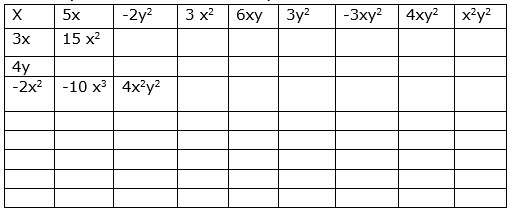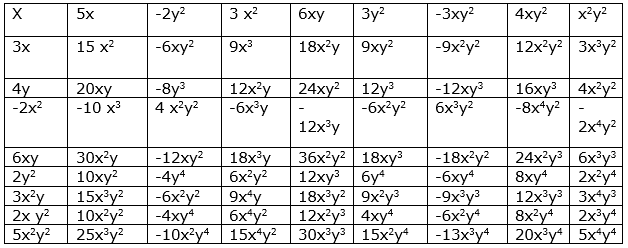Here the product is calculated in the following manner:

We multiply the terms on the x axis (horizontal)and y axis(vertical).

For example, when -2y2 is on the X axis (observe the 1st row 3rd column) and 3x is on the Y axis(2nd row 1st column) then ,the product

becomes -2y2×3x = -6xy2(observe the 2nd row 3rd column).

Question 7.

Find the volumes of rectangular boxes with given length, breadth and height in the following table.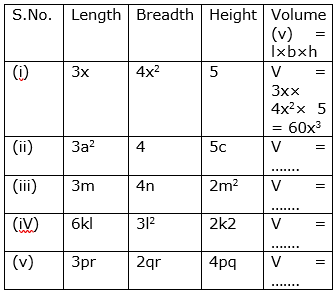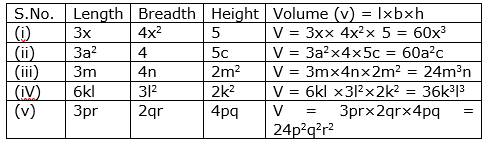Here we have calculated the Volume V using the Length, Breadth and Height.

Question 8.

Find the product of the following monomials

xy, x2y, xy, x

Here we have xy,x2y,xy,x

Hence the product of the above terms is (xy)×(x2y)×(x) =

(x.x2.x) (y.y)

= x4y2

Question 9.

Find the product of the following monomials

a, b, ab, a3b, ab3

Here we have a,b,ab,a3b,ab3

Hence the product of the above terms is (a)×(b)×(ab)×(a3b)×(ab3)

= (a.a.a3.a)(b.b.b.b3)

= a6b6

Question 10.

Find the product of the following monomials

kl, lm, km, klm

Here we have kl,lm,km,klm

Hence the product of the above terms is (kl)×(lm)×(km)×(klm)

= (k.k.k)(l.l.l)(m.m.m)

= k3l3m3

Question 11.

Find the product of the following monomials

pq ,pqr, r

Here we have pq,pqr,r

Hence the product of the above terms is (pq)×(pqr)×(r)

= (p.p)(q.q)(r.r)

= p2q2r2

Question 12.

Find the product of the following monomials

−3a, 4ab, −6c, d

Here we have -3a,4ab,-6c,d

Hence the product of the above terms is (-3a)×(4ab)×(-6c)×(d)

= (-3×4×-6)(a.a)(b)(c)(d)

= 72a2bcd

Question 13.

If A = xy, B = yz and C = zx, then find ABC = ...........

Here we have A = xy ,B = yz and C = zx.

Therefore the product of A,B and C will be ABC = (xy)×(yz)×(zx) = x2y2z2.

Here we have P = 4x2 ,T = 5x and R = 5y.

Therefore the product of P,T and R will be PTR = (4x2)×(5x)×(5y) = 100x3y

Hence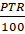is equal to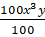i.e. x3y.

Question 15.

Write some monomials of your own and find their products.

The monomials are (i)5x,6y,7z and (ii)3x2y,4xy2,7x3y3

(i) The given monomial is 5x,6y,7z .

Therefore the product of above terms is (5x)×(6y)×(7z) = 210xyz

(ii) The given monomial is 3x2y,4xy2,7x3y3 .

Therefore the product of above terms is (3x2y)×(4xy2)×(7x3y3) = 84x6y6

###### Exercise 11.2

Question 1.

Complete the table: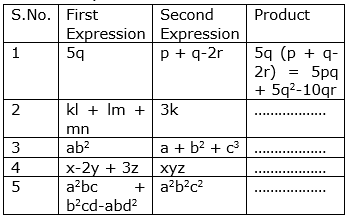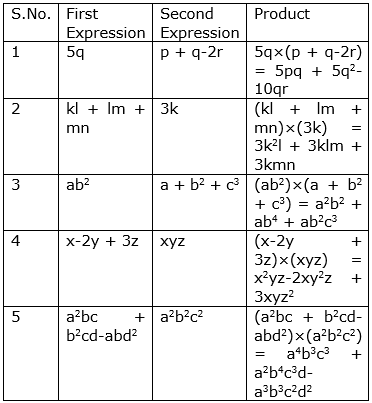Here we have the First and Second expressions as 5q and p + q-2r respectively.

The process involved in the multiplication is :

Step1.Write the product of the expressions using multiplication symbol: 5q×(p + q-2r)

Step2.Use distributive law :Multiply the monomial by the first term of the polynomial then multiply the monomial by the second term and then multiply the monomial by the third term of the polynomial and add their products: 5pq + 5q2-10qr

Step3.Simplify the terms: 5q2 + 5pq-10qr

2. Similarly ,following the above process we calculate

(kl + lm + mn)(3k)

= (3k)(kl) + (3k)(lm) + (3k)(mn)

= 3k2l + 3klm + 3kmn

3. Similarly ,following the above process we calculate

(ab2)( a + b2 + c3) = ab2(a) + ab2 (b2) + ab2(c3) = a2b2 + ab4 + ab2c3

4.Similarly ,following the above process we calculate

(x-2y + 3z)(xyz) = ( x-2y + 3z)×(xyz) = x2yz-2xy2z + 3xyz2

5. Similarly ,following the above process we calculate

(a2bc + b2cd-abd2)(a2b2c2) = (a2bc + b2cd- abd2)×(a2b2c2) = a4b3c3 + a2b4c3d-a3b3c2d2

Question 2.

Simplify: 4y(3y + 4)

Here we have 4y and 3y + 4.

Now the product of these terms is as follows:

The procedure involved in the multiplication is :

Step1.Write the product of the expressions using multiplication symbol: 4y(3y + 4).

Step2.Use distributive law :Multiply the monomial by the first term of the binomial then multiply the monomial by the second term of the binomial and then add their products: 4y(3y) + 4y(4)

Step3.Simplify the terms: 12y2 + 16y

Hence 4y(3y + 4) = 12y2 + 16y

Question 3.

Simplify x(2x2−7x + 3) and find the values of it for (i) x = 1 and (ii) x = 0

Here we have the monomial x and polynomial 2x2-7x + 3

Now the product of these terms is as follows:

The process involved in the multiplication is :

Step1.Write the product of the expressions using multiplication symbol:

x(2x2-7x + 3).

Step2.Use distributive law :Multiply the monomial by the first term of the trinomial then multiply the monomial by the second term of the trinomial and then the monomial by the third term of the trinomial and then add their products: x(2x2)-x(7x) + x(3)

Step3.Simplify the terms: 2x3-7x2 + 3x

Hence x(2x2−7x + 3) = 2x3-7x2 + 3x

(i)When x = 1, 2x3-7x2 + 3x = 2(1)3-7(1)2 + 3(1) = -2

(ii)When x = 0, 2x3-7x2 + 3x = 2(0)3-7(0)2 + 3(0) = 0

Question 4.

Add the product: a(a−b), b(b−c), c(c−a)

Here we have the expressions a(a-b),b(b-c) and c(c-a)

Using the distributive law a(a-b) = a2-ab,b(b-c) = b2-bc and c(c-a) = c2-ca

Now we add the terms (a2-ab) + (b2-bc) + (c2-ca)

= a2 + b2 + c2-ab-bc-ca

Hence addition of the product of the above expressions is a2 + b2 + c2-ab-bc-ca.

Question 5.

Add the product: x(x + y−r), y(x−y + r), z(x−y−z)

Here we have the expressions x(x + y-r),y(x-y + r) and z(x-y-z)

Using the distributive law x(x + y-r)

= xy-y2 + yr and

z(x-y-z) = xz-yz-z2

Now we add these terms (x2 + xy-xr) + (xy-y2 + yr) + (xz-yz-z2)

= x2-y2-z2 + 2xy-xr + yr + xz-yz

Hence addition of the product of the above expressions is x2-y2-z2 + 2xy-xr + yr + xz-yz.

Question 6.

Subtract the product of 2x(5x−y) from product of 3x(x + 2y)

Here we have 2x(5x-y) and 3x(x + 2y)

Using the distributive law 2x(5x-y) = 2x(5x)-2x(y)

= 10x2-2xy

and 3x(x + 2y)

= 3x(x) + 3x(2y)

= 3x2 + 6xy.

Now , we have to subtract 10x2-2xy from 3x2 + 6xy.

i.e. (3x2 + 6xy)-(10x2-2xy)

= 3x2 + 6xy-10x2 + 2xy

= -7x2 + 8xy

Hence after subtraction of the product of 2x(5x−y) from the product of 3x(x + 2y) is

-7x2 + 8xy.

Question 7.

Subtract 3k(5k−l + 3m) from 6k(2k + 3l−2m)

Here we have 3k(5k−l + 3m) and 6k(2k + 3l−2m)

Using the distributive law 3k(5k−l + 3m) = 15k2-3kl + 9km

and 6k(2k + 3l-2m) = 12k2 + 18kl-12km

Now , we have to subtract 15k2-3kl + 9km from 12k2 + 18kl-12km.

i.e. (12k2 + 18kl-12km)-(15k2-3kl + 9km) = -3k2 + 21kl-21k2m2

Hence after subtraction of the product of 3k(5k−l + 3m) from the product of 6k(2k + 3l−2m) is = -3k2 + 21kl-21k2m2

Question 8.

Simplify: a2(a−b + c) + b2(a + b−c)−c2(a−b−c)

Here we have the polynomials as a2(a−b + c), b2(a + b−c) and c2(a−b−c)

Now using the distributive law, a2(a−b + c) = a2(a)-a2(b) + a2(c) = a3-a2b + a2c

b2(a + b−c) = b2(a) + b2(b)-b2(c) = ab2 + b3-b2c and c2(a−b−c) = c2a-c2b-c3

Now we will simplify it, a2(a−b + c) + b2(a + b−c)−c2(a−b−c) = (a3-a2b + a2c) + (ab2 + b3-b2c)-(c2a-c2b-c3) = a3-a2b + a2c + ab2 + b3-b2c-c2a + c2b + c3 = a3 + b3 + c3-a2b + a2c + ab2-b2c-c2a + c2b

Hence , a2(a−b + c) + b2(a + b−c)−c2(a−b−c) = a2b + a2c + ab2-b2c-c2a + c2b

###### Exercise 11.3

Question 1.

Multiply the binomials:

2a−9 and 3a + 4

Consider two binomials as 2a−9 and 3a + 4

Now,to get the product of two binomials 2a−9 and 3a + 4 we use the

distributive law i.e. (2a−9)×(3a + 4) = 2a(3a) + 2a(4)-9(3a)-9(4) = 6a2 + 8a-27a-36 = 6a2-19a-36

Therefore ,the product of 2a−9 and 3a + 4 is 6a2-19a-36.

Question 2.

Multiply the binomials:

x−2y and 2x−y

Consider two binomials as x−2y and 2x−y

Now,to get the product of two binomials x−2y and 2x−y we use the

distributive law i.e. (x−2y)×( 2x−y) = x(2x)-x(y)-2y(2x)-2y(-y) = 2x2-xy-4xy + 4y2

Therefore ,the product of x−2y and 2x−y is 2x2 + 4y2-5xy.

Question 3.

Multiply the binomials:

kl + lm and k−l

Consider two binomials as kl + lm and k-l

Now,to get the product of two binomials kl + lm and k-l we use the

distributive law i.e. (kl + lm)×( k-l) = kl(k) + kl(-l) + lm(k) + lm(-l) = k2l-kl2 + klm-l2m

Therefore , the product of (kl + lm) and ( k-l) is k2l-kl2 + klm-l2m

Question 4.

Multiply the binomials:

m2−n2 and m + n

Consider two binomials as m2−n2 and m + n

Now, to get the product of two binomials ) m2−n2 and m + n we use the

distributive law i.e. (m2−n2)×( m + n) = m2(m) + m2(n)-n2(m)-n2(n) =

m3 + m2n-mn2-n3

Therefore , the product of m2−n2 and m + n is m3 + m2n-mn2-n3.

Question 5.

Find the product:

(x + y)(2x−5y + 3xy)

Consider the binomial x + y and the trinomial 2x−5y + 3xy.

Now, to get the product of above expressions x + y and 2x−5y + 3xy,we use the

distributive law i.e (x + y)×(2x−5y + 3xy) = x(2x-5y + 3xy) + y(2x-5y + 3xy)

= x(2x) + x(-5y) + x(3xy) + y(-5y) + y(3xy) = 2x2-5xy + 3x2y-5y2 + 3xy2

Therefore , the product of x + y and 2x−5y + 3xy is 2x2-5y2 + 3x2y + 3xy2-5xy

Question 6.

Find the product:

(mn−kl + km) (kl−lm)

Consider the trinomial mn−kl + km and the binomial kl−lm.

Now, to get the product of mn−kl + km and kl−lm,we use the

distributive law i.e. (mn−kl + km)×( kl−lm) = mn(kl-lm)-kl(kl-lm) + km(-lm)

= mnkl-m2nl-k2l2 + kl2m + kml-klm2

Therefore , the product of mn−kl + km and kl−lm is mnkl-m2nl-k2l2 + kl2m + kml-klm2

Question 7.

Find the product:

(a−2b + 3c)(ab2−a2b)

Consider the trinomial a−2b + 3c and the binomial ab2−a2b.

Now, to get the product of a−2b + 3c and ab2−a2b,we use the

distributive law i.e. (a−2b + 3c)×( ab2−a2b) = a(ab2-a2b)-2b(ab2-a2b) + 3c(ab2-a2b)

= a2b2-a3b-2ab3 + 2a2b2 + 3ab2c-3a2bc = 3a2b2-a3b-2ab3 + 3ab2c-3a2bc

Therefore , the product of a−2b + 3c and ab2−a2b is 3a2b2-a3b-2ab3 + 3ab2c-3a2bc

Question 8.

Find the product:

(p3 + q3)(p−5q + 6r)

Consider the binomial p3 + q3 and the trinomial p−5q + 6r.

Now, to get the product of p3 + q3 and p−5q + 6r,we use the

distributive law i.e (p3 + q3)(p−5q + 6r) = p3(p-5q + 6r) + q3(p-5q + 6r) = (p3.p-5p3q + p3.6r) + (p.q3-5q.q3 + 6q3r) = p4-5p3q + 6p3r + pq3-5q4 + 6q3r

Therefore , the product of p3 + q3and p−5q + 6r is p4-5p3q + 6p3r + pq3-5q4 + 6q3r

Question 9.

Simplify the following:

(x−2y)(y−3x) + (x + y)(x−3y)−(y−3x)(4x−5y)

Here we have (x−2y)(y−3x) + (x + y)(x−3y)−(y−3x)(4x−5y)

Re-arranging, we get,

(x - 2y)(y - 3x) - (y - 3x)(4x - 5y) + (x + y)(x - 3y)

= (y - 3x)[(x - 2y) - (4x - 5y)] + (x + y)(x - 3y)

= (y - 3x)(-3x + 3y) + (x + y)(x - 3y)

= - 3 (y - 3x)(x - y) + (x + y)(x - 3y)

= -3 (xy - y2 - 3x2 + 3xy) + x2 - 3xy + xy - 3y2= 9x2 + 3y2 - 12xy + x2 - 3y2 - 2xy

= 10x2 - 14xy

Question 10.

Simplify the following:

(m + n)(m2−mn + n2)

We have the binomial m + n and trinomial m2−mn + n2

Here we will use the distributive law as follows:

(m + n)(m2−mn + n2) = m(m2−mn + n2) + n(m2−mn + n2) = (m.m2-m.mn + m.n2) + (n.m2-mn.n + n.n2) = m3-m2n + mn2 + m2n-mn2 + n3 = m3 + n3

Hence , (m + n)(m2−mn + n2) = m3 + n3

Question 11.

Simplify the following:

(a−2b + 5c)(a−b)− (a−b−c)(2a + 3c) + (6a + b)(2c−3a−5b)

We have (a−2b + 5c)(a−b)− (a−b−c)(2a + 3c) + (6a + b)(2c−3a−5b)

Here, (a−2b + 5c)(a−b) = (a-b)(a-2b + 5c)(using Commutative law)

= a(a-2b + 5c)-b(a-2b + 5c)(using distributive law )

= a2-2ab + 5ac-ab + 2b2-5bc = a2 + 2b2-3ab + 5ac-5bc

(a−b−c)(2a + 3c) = (2a + 3c)(a-b-c) (using Commutative law)

= 2a(a-b-c) + 3c(a-b-c) (using distributive law )

= 2a2-2ab-2ac + 3ac-3bc-3c2

= 2a2 + ac-2ab-3bc-3c2

and (6a + b)(2c−3a−5b) = 6a(2c−3a−5b) + b(2c−3a−5b) = 12ac-18a2-30ab + 2bc-3ab-5b2 = -18a2 + 5b2-33ab + 2bc + 12ac

Therefore, a−2b + 5c)(a−b)− (a−b−c)(2a + 3c) + (6a + b)(2c−3a−5b) = a2 + 2b2-3ab + 5ac-5bc-(2a2 + ac-2ab-3bc-3c2) + ( -18a2 + 5b2-33ab + 2bc + 12ac) =

a2 + 2b2-3ab + 5ac-5bc-2a2-2ac + 2ab + 3bc + 3c2-18a2 + 5b2-33ab + 2bc + 12ac =

= -19a2 + 7b2 + 3c2-34ab + 15ac

Question 12.

Simplify the following:

(pq-qr + pr)(pq + qr)−(pr + pq)(p + q−r)

We have , (pq-qr + pr)(pq + qr)−(pr + pq)(p + q−r)

(pq-qr + pr)(pq + qr) = (pq + qr)( pq-qr + pr) (using Commutative law)

= pq(pq-qr + pr) + qr( pq-qr + pr) (using distributive law )

= p2q2-pq2r + p2qr + pq2r-q2r2 + pqr2 = p2q2 + p2qr + pqr2-q2r2

(pr + pq)(p + q−r) = pr(p + q-r) + pq(p + q−r) (using distributive law )

= p2r + prq-pr2 + p2q + pq2-prq

= p2r-pr2 + p2q + pq2

(pq-qr + pr)(pq + qr)−(pr + pq)(p + q−r) = p2q2 + p2qr + pqr2-q2r2-( p2r-pr2 + p2q + pq2)

= p2q2 + p2qr + pqr2-q2r2-p2r + pr2-p2q-pq2

Hence, (pq-qr + pr)(pq + qr)−(pr + pq)(p + q−r) = p2q2 + p2qr + pqr2-q2r2-p2r + pr2-p2q-pq2

Question 13.

If a, b, c are positive real numbers such that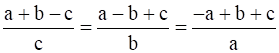, find the value of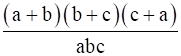Given: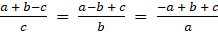, where a, b, c are positive real numbers

Here, we have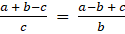⇒b(a + b-c) = c(a-b + c)

⇒ab + b2-bc = ac-bc + c2(bc is on both the sides and they get subtracted)

⇒ab + b2 = ac + c2

⇒ab-ac = c2-b2

⇒a(b-c) = (c-b)(c + b)

⇒-a(c-b) = (c + b)(c-b)

⇒-a = (c + b)[Here (c-b) is on both the sides,so when they get divided and the result is 1]

⇒(b + c) = -a(commutative property)

We have ,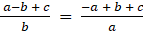⇒a(a-b + c) = b(-a + b + c)

⇒a2-ab + ac = -ab + b2 + bc

⇒a2 + ac = b2 + bc

⇒a2-b2 = bc-ac

⇒(a + b)(a-b) = -c(a-b)

⇒a + b = -c

We have ,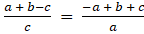⇒a(a + b-c) = c(-a + b + c)

⇒a2 + ab-ac = -ac + bc + c2

⇒a2 + ab = bc + c2

⇒a2-c2 = bc-ab

⇒(a + c)(a-c) = b(c-a)

⇒(a + c)(a-c) = -b(a-c)

⇒a + c = -b

Therefore,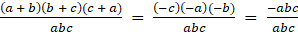= -1(Here we have substituted the values of (a + b),(b + c),(c + a) from above evaluation)

###### Exercise 11.4

Question 1.

Select a suitable identity and find the following products

(3k + 4l) (3k + 4l)

Given: (3k + 4l) (3k + 4l),it is a product of 2 binomial expressions which have the same terms 3k + 4l and 3k + 4l.

Now when we compare these expressions with the identities, we

find it in the form of (a + b)2,where a = 3k and b = 4l,

The identity (a + b)2 = a2 + 2ab + b2,

Hence, (3k + 4l) (3k + 4l) = (3k + 4l)2 = (3k)2 + 2(3k)(4l) + (4l)2 = 9k2 + 24kl + 16l2

Question 2.

Select a suitable identity and find the following products

(ax2 + by2) (ax2 + by2)

Given: (ax2 + by2) (ax2 + by2),it is a product of 2 binomial expressions which have the same terms ax2 + by2 and ax2 + by2.

Now when we compare these expressions with the identities, we

find it in the form of (a + b)2,where a = ax2 and b = by2,

The identity (a + b)2 = a2 + 2ab + b2,

Hence, (ax2 + by2) (ax2 + by2) = (ax2 + by2)2 = (ax2)2 + 2(ax2)(by2) + (by2)2

= a2x4 + 2abx2y2 + b2y4

Question 3.

Select a suitable identity and find the following products

(7d – 9e) (7d – 9e)

Given: (7d – 9e) (7d – 9e),it is a product of 2 binomial expressions which have the same terms 7d – 9e and 7d – 9e.

Now when we compare these expressions with the identities, we

find it in the form of (a-b)2,where a = 7d and b = 9e,

The identity (a-b)2 = a2-2ab + b2,

Hence, (7d – 9e) (7d – 9e) = (7d-9e)2 = (7d)2-2(7d)(9e) + (9e)2 = 49d2-126de + 81e2

Question 4.

Select a suitable identity and find the following products

(m2 – n2) (m2 + n2)

Given:( m2 – n2) (m2 + n2),it is a product of 2 binomial expressions which have the terms (m2 – n2) and (m2 + n2).

Now when we compare these expressions with the identities, we

find it in the form of (a-b)(a + b),where a = m2 and b = n2,

The identity (a-b)(a + b) = a2-b2,

Hence, (m2 – n2) (m2 + n2) = (m2)2-(n2)2 = m4-n4

Question 5.

Select a suitable identity and find the following products

(3t + 9s) (3t – 9s)

Given:(3t + 9s) (3t – 9s),it is a product of 2 binomial expressions which have the terms :(3t + 9s) and (3t – 9s).

Now when we compare these expressions with the identities, we

find it in the form of (a-b)(a + b),where a = 3t and b = 9s,

The identity (a-b)(a + b) = a2-b2,

Hence, (3t + 9s) (3t – 9s) = (3t)2-(9s)2 = 9t2-81s2

Question 6.

Select a suitable identity and find the following products

(kl – mn) (kl + mn)

Given:( kl – mn) (kl + mn),it is a product of 2 binomial expressions which have the terms :( kl – mn) and (kl + mn).

Now when we compare these expressions with the identities, we

find it in the form of (a-b)(a + b),where a = kl and b = mn,

The identity (a-b)(a + b) = a2-b2,

Hence, (kl – mn) (kl + mn) = (kl)2-(mn)2

Question 7.

Select a suitable identity and find the following products

(6x + 5) (6x + 6)

Given:(6x + 5) (6x + 6),it is a product of 2 binomial expressions which have the terms (6x + 5) and (6x + 6).

Now when we compare these expressions with the identities, we

find it in the form of (x + a)(x + b),where x = 6x and a = 5 and b = 6,

Thus,(x + a)(x + b) = x2 + x(b + a) + ab

Hence, (6x + 5) (6x + 6) = (6x)2 + 6x(5 + 6) + 30 = 12x2 + 66x + 30

Question 8.

Select a suitable identity and find the following products

(2b – a) (2b + c)

Given: (2b – a) (2b + c),it is a product of 2 binomial expressions which have the terms (2b – a) (2b + c).

Now when we compare these expressions with the identities, we

find it in the form of (x-a)(x + b),where x = 2b and a = a and b = c,

Thus,(x-a)(x + b) = x2 + bx-ax-ab = x2 + x(b-a)-ab

Hence, (2b – a) (2b + c) = (2b)2 + 2b(c-a)-ac = 4b2 + 2b(c-a)-ac

Question 9.

Evaluate the following by using suitable identities:

3042

Given:3042 = (300 + 4)2 = (300)2 + 2(300)(4) + (4)2

= 90000 + 2400 + 16, where a = 300 and b = 4 in the identity (a + b)2 = a2 + 2ab + b2

= 92416

Question 10.

Evaluate the following by using suitable identities:

5092

Given: 5092 = (500 + 9)2 = (500)2 + 2(500)(9) + (9)2

= 2500 + 9000 + 81, where a = 500 and b = 9 in the identity (a + b)2 = a2 + 2ab + b2

= 11581

Question 11.

Evaluate the following by using suitable identities:

9922

Given: 9922 = (900 + 2)2 = (900)2 + 2(900)(2) + (2)2

= 8100 + 3600 + 4, where a = 900 and b = 2 in the identity (a + b)2 = a2 + 2ab + b2

= 11704

Question 12.

Evaluate the following by using suitable identities:

7992

Given: 7992 = (800-1)2 = (800)2-2(800)(1) + (1)2

= 6400 + 1600 + 1, where a = 800 and b = 1 in the identity (a-b)2 = a2-2ab + b2

= 8001

Question 13.

Evaluate the following by using suitable identities:

304 × 296

Given: 304×296 = (300 + 4)(300-4)

= (300)2-(4)2, where a = 300 and b = 4 in identity (a + b)(a-b) = a2-b2

= 90000-16 = 89984

Question 14.

Evaluate the following by using suitable identities:

83 × 77

Given:83×77 = (80 + 3)(80-3)

= (80)2-(3)2,where a = 80 and b = 3 in identity (a + b)(a-b) = a2-b2

= 6400-9 = 6391

Question 15.

Evaluate the following by using suitable identities:

109×108

Given:109×108 = (100 + 9)(100 + 8)

= (100)2 + (9 + 8)100 + (9×8),

where x = 100,a = 9 and b = 8 in identity (x + a)(x + b) = x2 + (a + b)x + ab

= 10000 + 1700 + 72

= 11772

Question 16.

Evaluate the following by using suitable identities:

204×206

Given: (200 + 4)(200 + 6)

= (200)2 + (4 + 6)200 + (4×6),

where x = 200,a = 4,b = 6 in identity (x + a)(x + b) = x2 + (a + b)x + ab

= 40000 + 2000 + 24

= 42024

###### Exercise 11.5

Question 1.

Verify the identity (a + b)2 ≡ a2 + 2ab + b2 geometrically by taking

a = 2 units, b = 4 units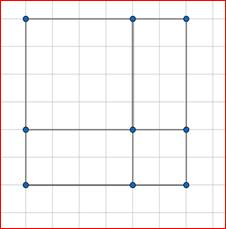(a + b)2Ξa2 + 2ab + b2

Draw a square with the side a + b i.e.,2 + 4

L.H.S of the whole square = (2 + 4)2 = (6)2 = 36

R.H.S = Area of the square with 2 units + Area of the square with 4 units +

Area of the 2,4 units + Area of the square with 4 ,2 units = 22 + 42 + 2×4 + 2×4 = 4 + 16 + 8 + 8 = 36

L.H.S = R.H.S

Hence,the identity is verified.

Question 2.

Verify the identity (a + b)2 ≡ a2 + 2ab + b2 geometrically by taking

a = 3 units, b = 1 unit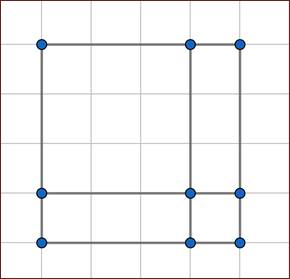(a + b)2Ξa2 + 2ab + b2

Draw a square with the side a + b i.e.,3 + 1

L.H.S of the whole square = (3 + 1)2 = (4)2 = 16

R.H.S = Area of the square with 3 units + Area of the square with 1 unit +

Area of the 3,1 unit + Area of the square with 1 ,3 units = 32 + 12 + 3×1 + 1×3 = 9 + 1 + 3 + 3 = 16

L.H.S = R.H.S

Hence, the identity is verified.

Question 3.

Verify the identity (a + b)2 ≡ a2 + 2ab + b2 geometrically by taking

a = 5 units, b = 2 unit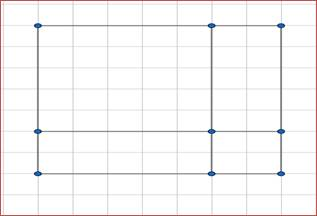(a + b)2Ξa2 + 2ab + b2

Draw a square with the side a + b i.e.,5 + 2

L.H.S of the whole square = (5 + 2)2 = (7)2 = 49

R.H.S = Area of the square with 5 units + Area of the square with 2 units +

Area of the 5,2 units + Area of the square with 2 ,5 units = 52 + 22 + 5×2 + 2×5 = 49

L.H.S = R.H.S

Hence,the identity is verified.

Question 4.

Verify the identity (a – b)2 ≡ a2 - 2ab + b2geometrically by taking

a = 3 units, b = 1 unit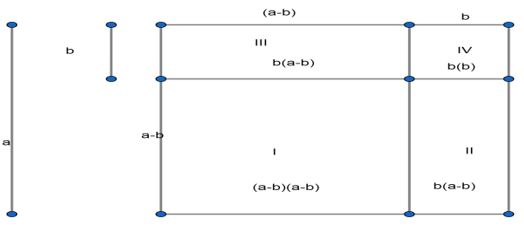(a – b)2 ≡ a2 - 2ab + b2

Consider a square with side a.i.e.a = 3

The square is divided into 4 regions.

It consists of 2 squares with sides a-b and b respectively and 2 rectangles with length and breadth as ‘a-b’ and ‘b’ respectively.

Here a = 3 and b = 1.Therefore the 2 squares consist of sides ‘3-1’ and ‘1’ respectively and 2 rectangles with length and breadth as ‘3-1’ and ‘1’ respectively.

Now area of figure I = Area of whole square with side ‘a’ i.e.3 units-Area of figure II

-Area of figure III –Area of figure IV

L.H.S of area of figure I = (3-1)(3-1) = 2(2) = 4 units

R.H.S = Area of whole square with side 3 units-Area of figure II with 1,(3-1)units

-Area of figure III with 1,(3-1) units –Area of figure IV with 1,1 unit = 32-(1×(3-1))-

(1×(3-1))-(1×1) = 4 units

L.H.S = R.H.S

Hence,the identity is verified.

Question 5.

Verify the identity (a – b)2 ≡ a2 - 2ab + b2geometrically by taking

a = 5 units, b = 2 units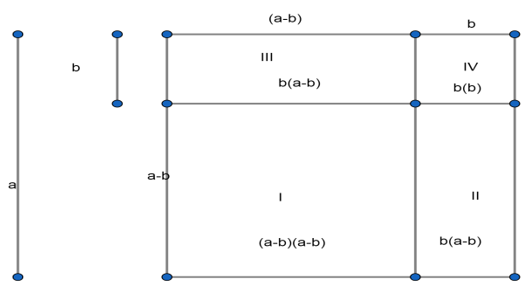(a – b)2 ≡ a2 - 2ab + b2

Consider a square with side a.i.e.a = 5

The square is divided into 4 regions.

It consists of 2 squares with sides a-b and b respectively and 2 rectangles with length and breadth as ‘a-b’ and ‘b’ respectively.

Here a = 5 and b = 2.Therefore the 2 squares consist of sides ‘5-2’ and ‘2’ respectively and 2 rectangles with length and breadth as ‘5-2’ and ‘2’ respectively.

Now area of figure I = Area of whole square with side ‘a’ i.e.5 units-Area of figure II

-Area of figure III –Area of figure IV

L.H.S of area of figure I = (5-2)(5-2) = 3(3) = 9 units

R.H.S = Area of whole square with side 5 units-Area of figure II with 2,(5-2)units

-Area of figure III with 2,(5-2) units –Area of figure IV with 2,2 units = 25-(2×3)-

( 2×3)-22 = 25-6-6-4 = 9 units

L.H.S = R.H.S

Hence,the identity is verified.

Question 6.

Verify the identity (a + b) (a – b) ≡ a2 – b2 geometrically by taking

a = 3 units, b = 2 units

Remove square from this whose side is ‘b’.(b<a)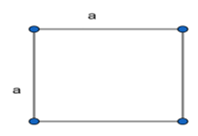Consider a square with side ‘a’.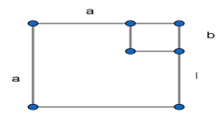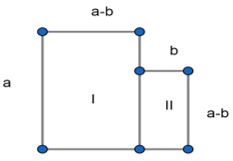We get the above figure by removing the square with side ‘b’.It consists of 2 parts

I and II.

So a2-b2 = Area of figure I + Area of figure II = a(a-b) + b(a-b) = (a-b)(a + b)

Thus a2-b2 = (a-b)(a + b).

Here a = 3 units and b = 2 units

So,L.H.S = a2-b2 = 32-22 = 5 units

R.H.S = (a-b)(a + b) = (3-2)(3 + 2) = 1(5) = 5 units

Therefore L.H.S = R.H.S

Hence,the identity is verified.

Question 7.

Verify the identity (a + b) (a – b) ≡ a2 – b2 geometrically by taking

a = 2 units, b = 1 unit

Refer the above figure.

Here a2-b2 = Area of figure I + Area of figure II = a(a-b) + b(a-b) = (a-b)(a + b)

Thus a2-b2 = (a-b)(a + b).

Here a = 2 units and b = 1 units

So, L.H.S = a2-b2 = 22-12 = 3 units

R.H.S = (a-b)(a + b) = (2-1)(2 + 1) = 3 units

Therefore L.H.S = R.H.S

Hence,the identity is verified.

## TGAREIA WEBSITE MAINTED BYTop